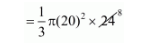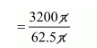# Water flows at the rate of 10 metre per minute`
Question:

Water flows at the rate of 10 metre per minute from a cylindrical pipe 5 mm in diameter. How long will it take to fill up a conical vessel whose diameter at the base is 40 cm and depth 24 cm?

(a) 48 minutes 15 sec

(b) 51 minutes 12 sec

(c) 52 minutes 1 sec

(d) 55 minutes

Solution:

$r=5 / 2 \mathrm{~mm}=0.25 \mathrm{~cm}$

The volume per minute of water flow from the pipe

$=\pi \times(0.25)^{2} \times 1000$

$=62.5 \pi \mathrm{cm}^{3} /$ minute

$=\frac{40}{2}$

$=20 \mathrm{~cm}$

Depth of cone = 24 cm

The volume of cone$=3200 \pi \mathrm{cm}^{3}$

The time it will take to fill up a conical vessel$=51 \frac{125}{625} \mathrm{~min}$

$=51 \mathrm{~min}+\frac{125}{625} \times 60 \mathrm{sec}$

$=51 \mathrm{~min}+12 \mathrm{sec}$

Hence, the correct answer is choice (b).